Technical Article

# Negative Feedback Part 3: Improving Noise, Linearity, and Impedance

November 13, 2015 by Robert Keim

## Use negative feedback to increase your amplifier’s signal-to-noise ratio, reduce its nonlinear distortion, and improve its input- and output-impedance characteristics.

You can use negative feedback to increase your amplifier’s signal-to-noise ratio, reduce its nonlinear distortion, and improve its input- and output-impedance characteristics.

### Supporting Information

Just so you don’t have to switch pages every time you want to ponder the general feedback structure, here is the diagram presented in the first article: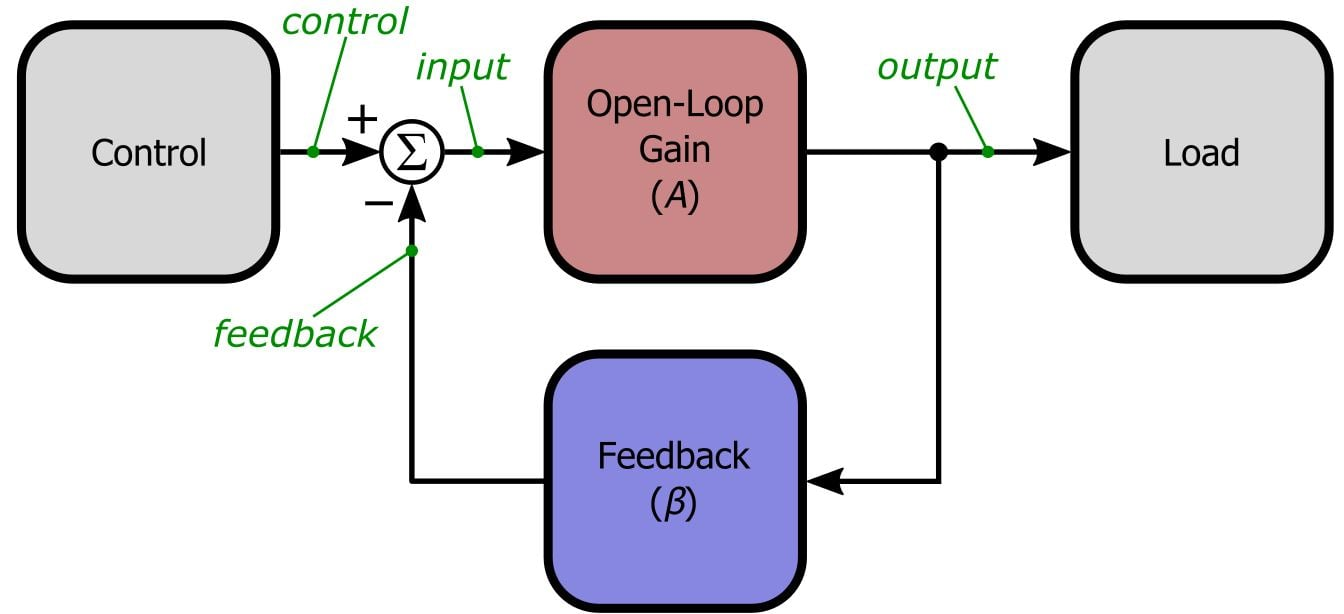### The Lesser-Known Benefits

It is difficult to be completely unaware of the negative-feedback benefits discussed in the previous article. Even if you have not studied the theoretical nature of gain desensitization and bandwidth extension, you probably know from your experience with op-amps that negative-feedback amplifier circuits are 1) not significantly affected by the open-loop gain of the particular op-amp being used and 2) governed by a gain-bandwidth product that tells you whether the op-amp can provide sufficient bandwidth at your chosen closed-loop gain. But to make this discussion more comprehensive, we need to look at three other ways in which negative feedback can significantly improve amplifier performance.

These beneficial properties, however, are somewhat more subtle and specialized than the first two. Why? Well, because nowadays all interaction between amplifiers and engineers is dominated by op-amps, and these other three benefits are not so directly or universally applicable to standard op-amp circuits; the reasons for this will become more clear as the article progresses.

We usually think of feedback amplifiers as increasing the amount of noise in a circuit—after all, op-amps suffer from input voltage noise and input current noise, and external resistors create Johnson noise. Nevertheless, in specialized situations we can increase the signal-to-noise ratio (SNR) at the output of an existing amplifier by adding another amplifier and a generous dose of negative feedback. The specialized situation is this: if you start with a noisy (and typically low-voltage-gain) amplifier, you can place a low-noise, high-voltage-gain amplifier in front of the noisy amplifier and apply negative feedback around both stages. The result is a significant improvement in SNR.

Here is a visual representation of the initial scenario: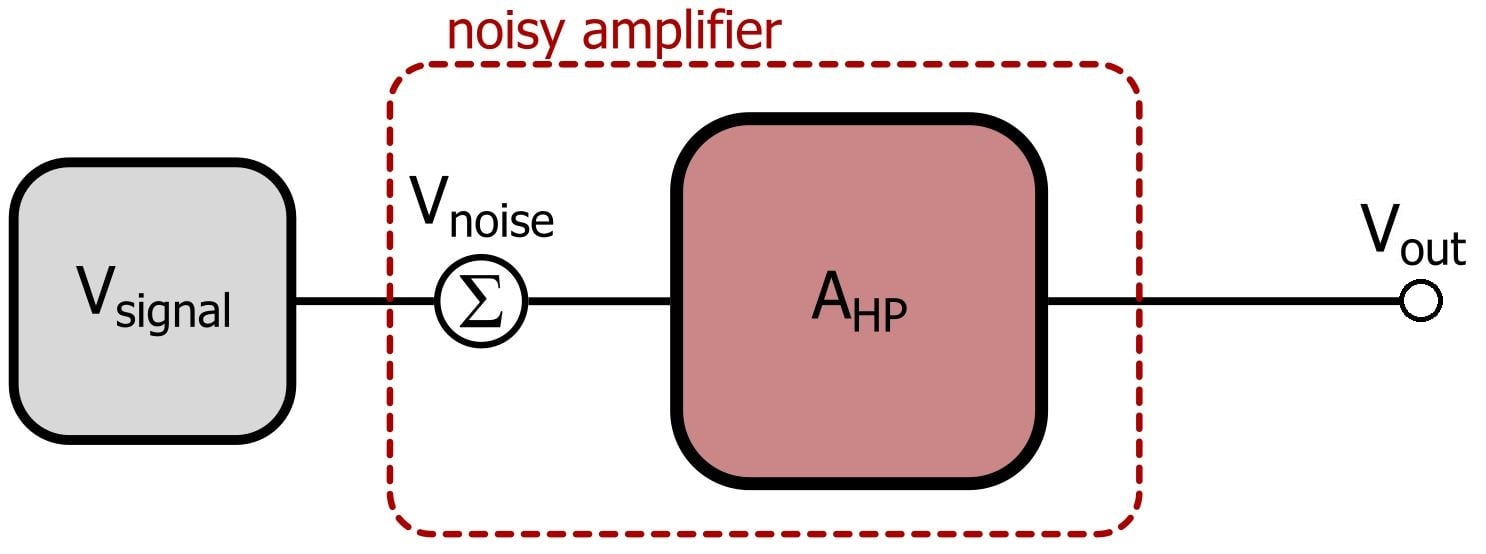As you can see, we have modeled the noisy amplifier as a gain AHP in series with a noise voltage that is added to the input signal. We refer to the gain as AHP because a typical application of this noise-reduction technique involves a high-power output amplifier preceded by a low-noise preamplifier (denoted by ALN). From the above diagram we see that the SNR of the input signal is Vsignal/Vnoise, and since both terms are amplified equally by AHP, the output SNR is also Vsignal/Vnoise.

$SNR=\frac{V_{signal}}{V_{noise}}$

Now we need a low-noise amplifier in front of the noisy amplifier. I know what you’re thinking: for goodness’ sake, why not just choose a low-noise amplifier in the first place? Well, it turns out that it is difficult (or expensive) to maintain good noise performance with amplifiers that draw large amounts of current from their (perhaps poorly filtered) high-wattage power supplies. Thus, this feedback-based noise-reduction technique is essentially a way of using the superior noise performance of low-power amplifiers to reduce the effects of noise introduced by a high-power amplifier. So here is the new circuit model: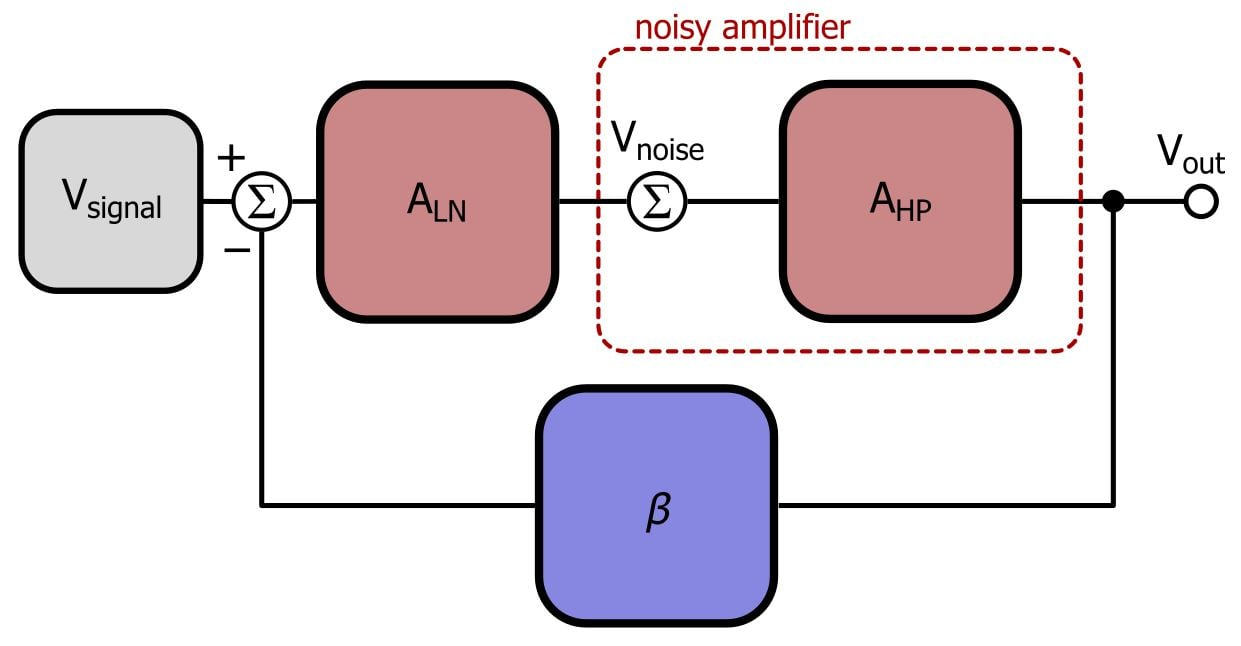Recall our formula for the closed-loop gain of a negative-feedback amplifier:

$G_{CL}=\frac{A}{1+A\beta}$

The loop gain (corresponding to in the generic formula) for the new circuit model is ALNAHPβ, and we need to further modify the input-to-output relationship to account for the fact that Vsignal is multiplied by ALN and AHP, but Vnoise is multiplied only by AHP. This is the result:

$V_{out}=V_{signal}\left(\frac{A_{LN}A_{HP}}{1+A_{LN}A_{HP}\beta}\right)+V_{noise}\left(\frac{A_{HP}}{1+A_{LN}A_{HP}\beta}\right)$

The important thing to take away from this equation is that the output voltage includes both the noise signal and the original input signal, but the signal component is larger than the noise component by the factor ALN. Thus, the ratio of signal to noise has been multiplied by ALN.

$SNR_{new}=\frac{V_{signal}}{V_{noise}}\times A_{LN}$

This is a significant result because with little trouble and expense we can greatly increase the SNR; keep in mind, though, that the gain of the preamp cannot be so high as to cause the signal to exceed the input-voltage limits established by the power amp’s supply voltages. Also, the power-amplifier stage represented by AHP usually has a voltage gain of unity; its purpose is to supply high current to the load, not to increase the voltage amplitude. Consequently, we would choose β = 1 to achieve SNR improvement while maintaining the appropriate overall gain.

### Dealing with Distortion

An ideal amplifier provides consistent gain for any input signal. We have already seen that real-life amplifiers eventually lose gain as the frequency of the input increases. Another standard characteristic of practical amplifier circuits is nonlinear distortion, which is a result of the fact that nonideal amplifiers exhibit different amounts of gain for input signals of different amplitude. We won’t go into mathematical details for this topic, but we can nonetheless conclude from intuition that negative feedback will reduce an amplifier’s nonlinear distortion: if feedback results in a closed-loop response that is only partially dependent on the amplifier’s open-loop gain and frequency characteristics, it follows that the closed-loop response will be only partially influenced by the amplifier’s distortion characteristics.

So adding negative feedback to an amplifier actually reduces the amount of nonlinear distortion in the output signal. If you find this surprising or novel, remember what we said at the beginning of the article regarding these “lesser-known benefits”: they aren’t directly or universally applicable to op-amp circuits. In the case of distortion, we don’t think about the influence of feedback because it is assumed that the op-amp will always be used in a feedback configuration. In other words, the distortion of the op-amp itself is largely irrelevant. This fact is evident in the following plot for the AD8044 amplifier from analog devices: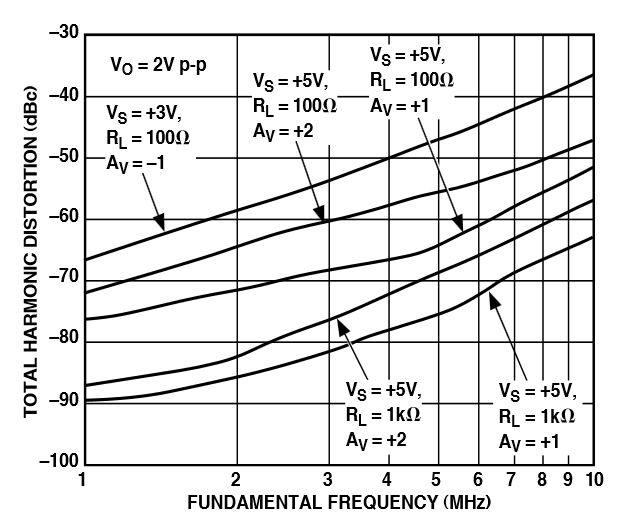As you can see, the distortion specs are given for a variety of closed-loop gains, but not for the open-loop gain.

The following circuits demonstrate what nonlinear distortion is and a straightforward way in which negative feedback can reduce it. The first circuit is a unity-gain op-amp driving a class B output stage.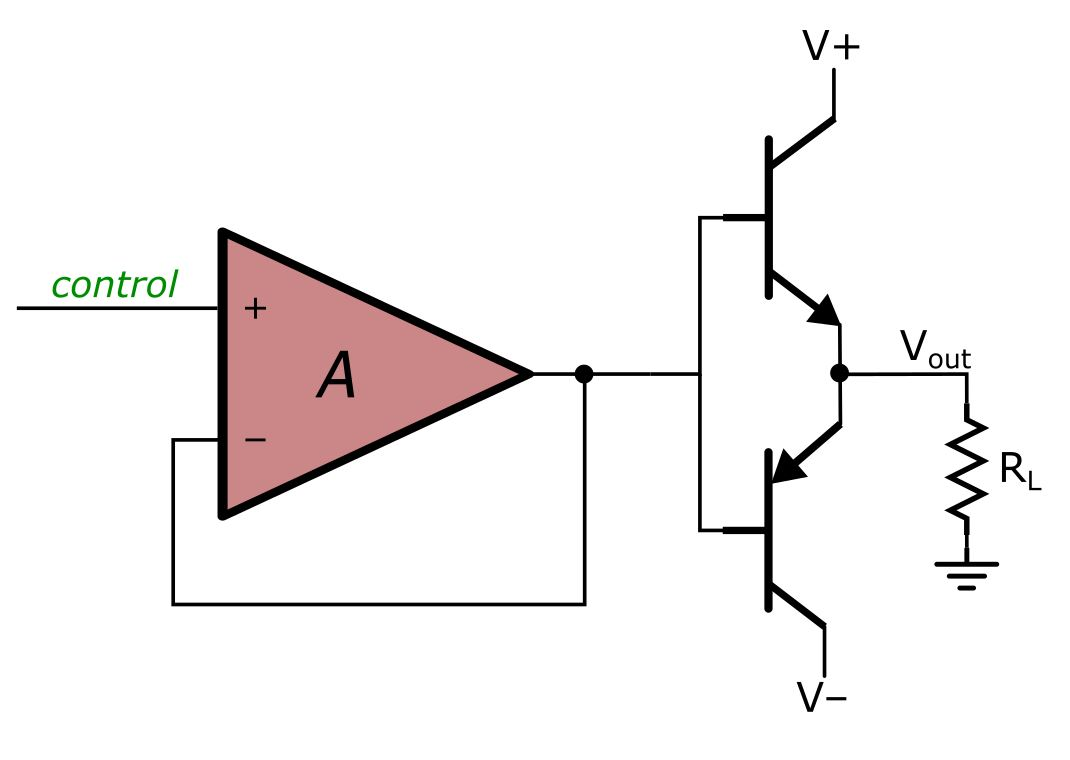Now take a look at the output voltage as the input signal increases from -3 V to +3 V.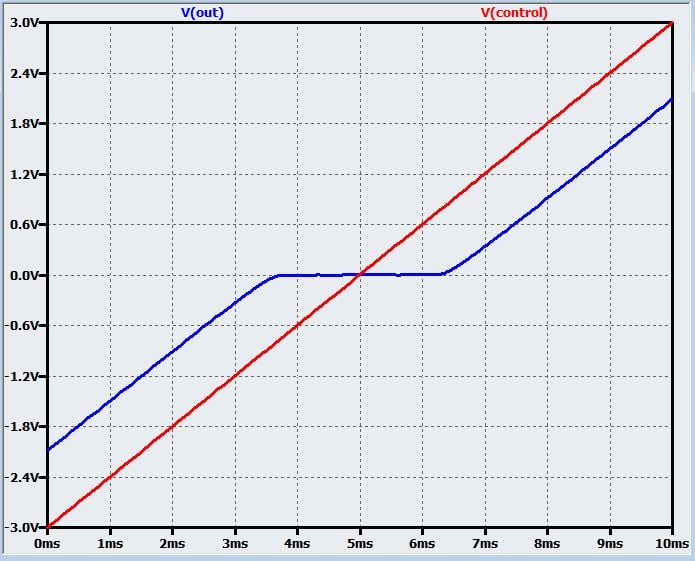The input-to-output relationship is consistent except for the dead band, where the control voltage is not high enough to turn on the NPN transistor or low enough to turn on the PNP transistor. This is an example of distortion, because the gain changes according to the amplitude of the input signal. We can almost completely eliminate this distortion by incorporating negative feedback from the output of the class B stage: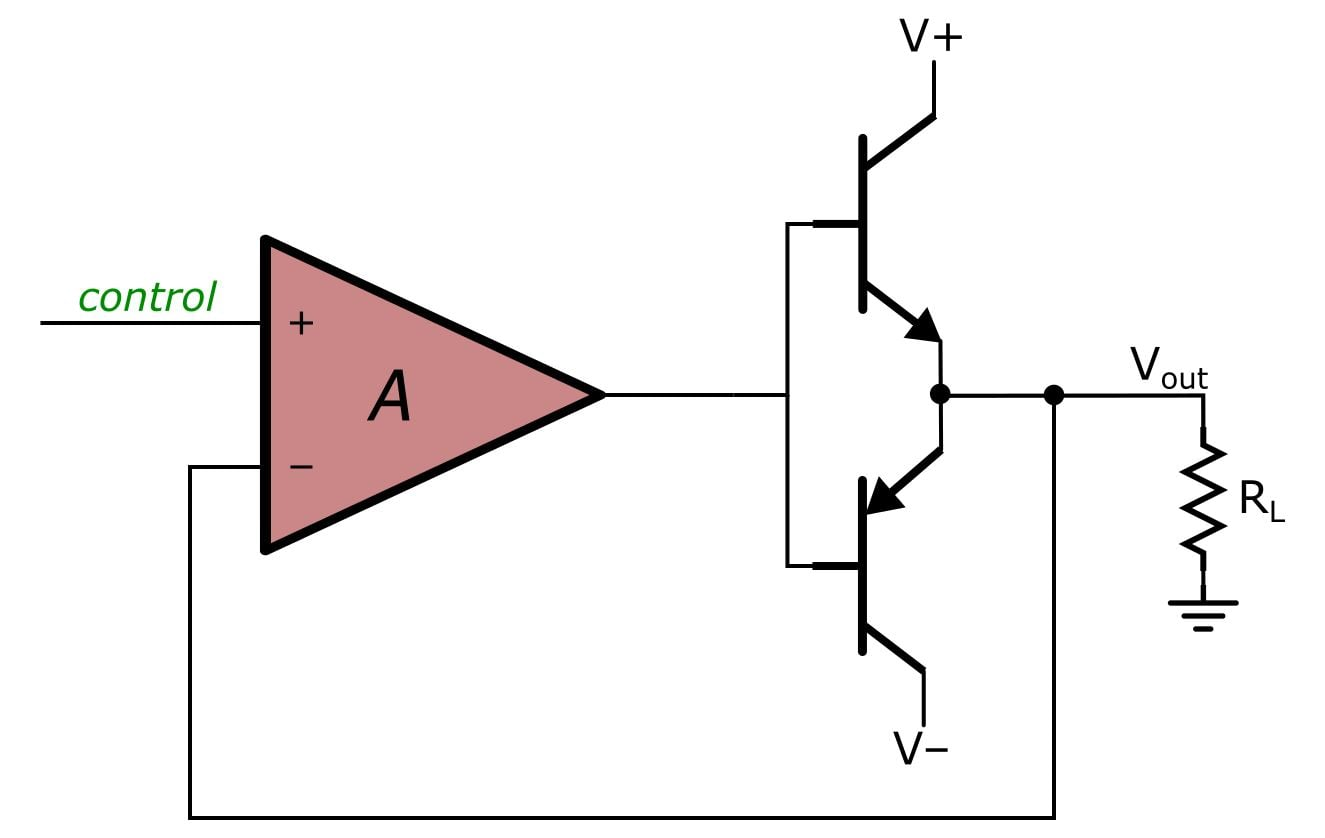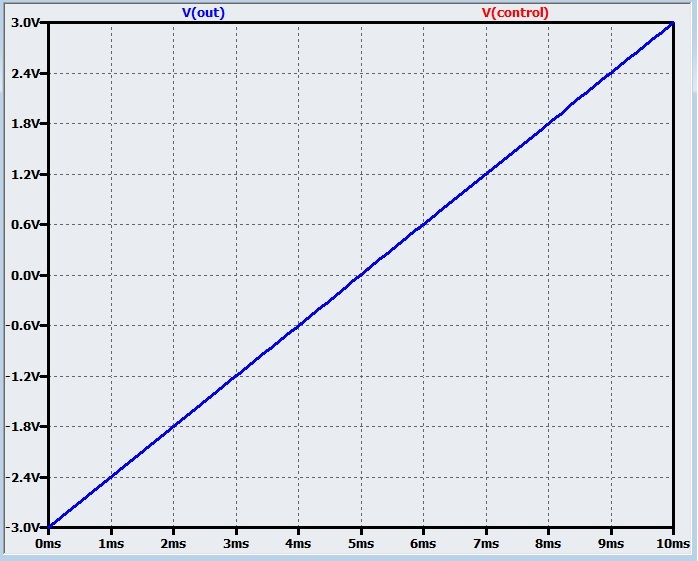You can’t see the Vcontrol trace because it is exactly underneath the Vout trace. Thus, negative feedback has not only eliminated the distortion but also removed the offset (caused by the transistors’ base-to-emitter voltage drop) between control and output.

### Improving Impedance . . . Sometimes

In general, an amplifier should have high input impedance and low output impedance. This ensures that minimal voltage is dropped across the impedance of the circuit driving the amplifier and that most of the output voltage is delivered to the load. By now it should come as no surprise that negative feedback can both increase input impedance and reduce output impedance. Perhaps you will again be unsurprised when you find out that the factor by which impedance is increased or reduced is a familiar quantity: (1 + ). Imagine for a moment that we decided to use an op-amp as an open-loop amplifier.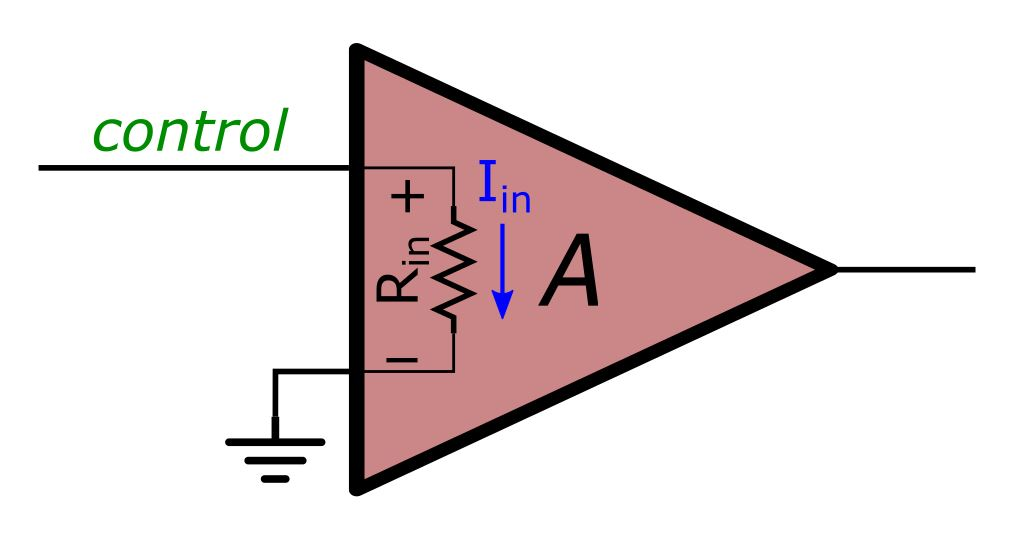The input impedance is calculated as (voltage at positive input terminal) – (voltage at negative input terminal) divided by input current:

$R_{in}=\frac{V_{in+}-V_{in-}}{I_{in}}$

In this case the voltage at the negative input terminal is 0 V, and the effective input impedance is simply the op-amp’s open-loop input impedance RinOL. Now imagine that we switch to a closed-loop configuration: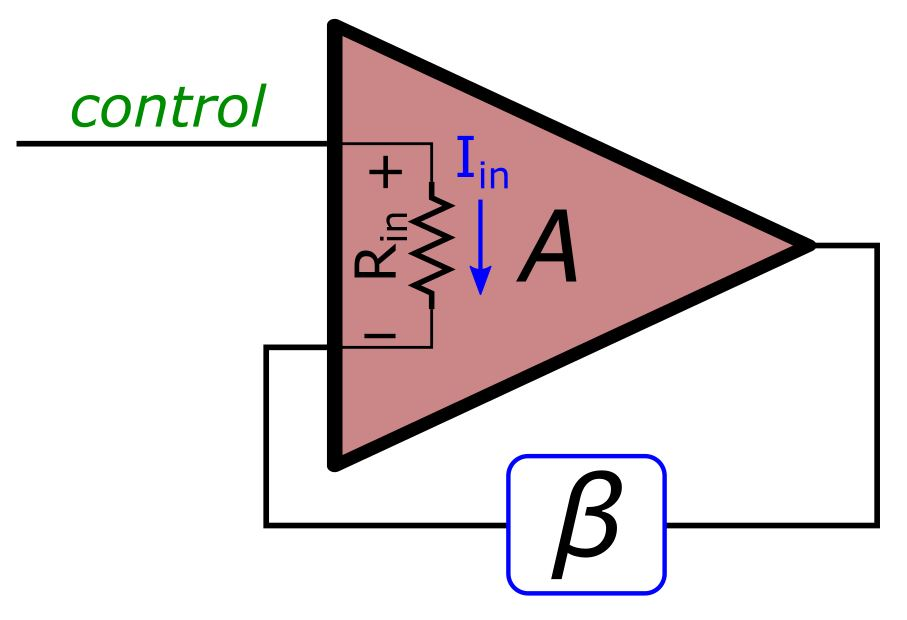The voltage at the negative terminal is no longer 0 V but rather (Vin+ × GCL) × β:

$I_{in}=\frac{V_{in+}-\left(V_{in+}\left(\frac{\beta A}{1+A\beta}\right)\right)}{R_{inOL}}=\frac{V_{in+}\left(1-\left(\frac{\beta A}{1+A\beta}\right)\right)}{R_{inOL}}$

Hence,

$\frac{V_{in+}}{I_{in}}=\frac{R_{inOL}}{1-\left(\frac{\beta A}{1+A\beta}\right)}=\frac{R_{inOL}}{\left(\frac{1+A\beta}{1+A\beta}\right)-\left(\frac{\beta A}{1+A\beta}\right)}=\frac{R_{inOL}}{\frac{1}{1+A\beta}}$

Thus, the effective input impedance with feedback (RinFB) is

$\frac{V_{in}}{I_{in}}=R_{inFB}=R_{inOL}\left(1+A\beta\right)$

A similar (though somewhat more complicated analysis) can demonstrate that the output impedance decreases by the same factor:

$R_{outFB}=\frac{R_{outOL}}{1+A\beta}$

One last important note: The astute reader may be wondering why the standard inverting op-amp circuit is noted for its low input impedance—what happened to negative feedback being beneficial for impedance characteristics? Well, there are actually four negative-feedback topologies, and this impedance analysis does not apply to all four. It does apply to the “series–shunt” topology, of which the standard noninverting op-amp circuit is an example. However, it does not apply to the standard inverting op-amp circuit, which belongs to the “shunt–shunt” topology.

### Conclusion

We have now expounded quite satisfactorily upon the benefits that negative feedback can confer on an amplifier circuit. In the next article we will explore an even more invigorating topic: stability.

Next Article in Series: Negative Feedback, Part 4: Introduction to Stability